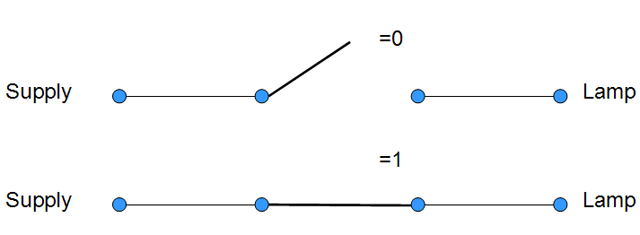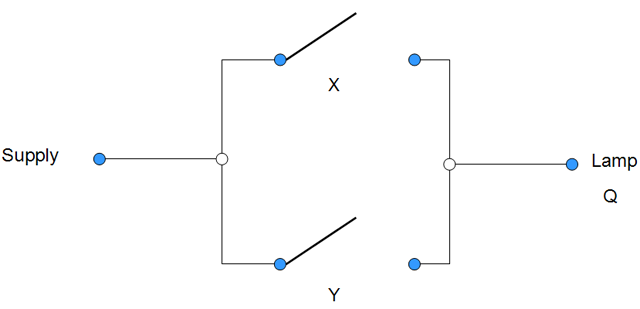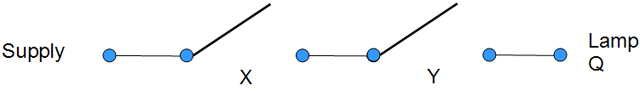# Computer ScienceLogic Gates - Truth Tables

The term 'Boolean' derives from the surname of the 19th century mathematician, George Boole. His shorthand notation for logic was based on theories set forth by Aristotle and others.

In short, a boolean variable can hold one of two values, true or false. Often, these variables are recast to 1 and 0 in computers.

We can see how these boolean values are represented in a simple circuit in the following diagram,When the circuit is completed, we get the boolean value of 1, when it is not complete, we get a value of 0.

## The OR FunctionThe OR function has two inputs, X & Y. The output for each combination of inputs is shown in the truth table below.

XYQ
0 0 0
0 1 1
1 0 1
1 1 1

The OR function returns a 1 if either or both of the inputs are 1.

The Boolean equation for this circuit describes the output Q in terms of the inputs, X and Y. We use the addition sign to indicate OR.

X + Y = Q

## The AND FunctionThe AND function takes two inputs and produces an output based on those values.

The truth table is as follows.

XYQ
0 0 0
0 1 0
1 0 0
1 1 1

The Boolean equation for this circuit describes the output Q in terms of the inputs, X and Y. We use the full stop to indicate AND.

X.Y = Q

## The NOT Function

The NOT function takes a single input. The output is always the inverse of the input.

YQ
0 1
1 0

We draw a bar over any variable that is passed through a NOT gate.

X = Q

## Other Functions

The three functions that you have seen so far are the fundamental functions. By combining the circuits that represent these functions, we can create some ones which are also useful to us.

Three other functions that you will meet are,

• Exclusive Or (XOR)
• NAND
• NOR

## XOR

XYQ
0 0 0
0 1 1
1 0 1
1 1 0

The XOR function takes two inputs and returns true if either (but not both) are set to 1. The boolean equation for this is,

A.B + A.B = Q

## NAND

The NAND function is a combination of the AND & NOT functions. It's truth table is as follows,

XYQ
0 0 1
0 1 1
1 0 1
1 1 0

Since NAND means NOT AND, we only get an output of false if both inputs are true. The boolean expression for this function is,

A.B = Q

## NOR

The NOR function is a combination of the OR & NOT functions. It's truth table is as follows,

XYQ
0 0 1
0 1 0
1 0 0
1 1 0

Since NOR means NOT OR, we only get an output of false if both inputs are false. The boolean expression for this function is,

A + B = Q